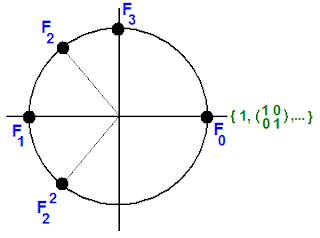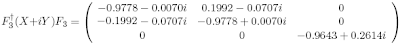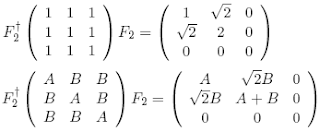occasional meanderings in physics' brave new world

Friday, October 31, 2008

Go Tumbling DownThis is the image brought to my mind by today's arxiv news. Unfortunately, I expect this to generate an ocean of sparticly papers, and only on their rotting pulp will the cards finally fall.

Update: Here is Tommaso's informed post on the subject, and here is Carl's.

M Theory Lesson 235

Once we have selected two different bases in the vector space $\mathbb{C}^{n}$, there is a discrete Fourier transform map between them, represented by an $n \times n$ matrix. For example, when $n = 3$, the Fourier arrows form a triangle. In general, they form an $n$-simplex.

Recall that a simplicial set is a functor from the collection of ordinals $N$, where each $N$ is drawn as an $N+1$-simplex, into the category Set. In other words, the functor first picks out $n$ sets to put at the vertices of each $n$-simplex, for all $n$. We would like to replace the ordinals by MUB simplices. This is natural, because at a fixed $n$ the vertices are labelled by q-numbers of dimension $n$. For example, the MUB triangle of $2 \times 2$ Pauli operators replaces the set $\{ 0,1,2 \}$ of the classical ordinal 2.

This construction uses numbers belonging to finite dimensional vector spaces, rather than sets. But in M Theory it is OK to think of the category of vector spaces as a quantum analogue of Set, and in quantum physics, why would we wish to calculate using classical numbers?

Thursday, October 30, 2008

Quick Update III

I went down to the hot pools for the first time in a while this morning. There was a cool sou'west breeze and a little high cloud to block out the dangerous sun. Meanwhile, I seem to be applying for a work permit overseas. Who knows what will happen next?

Sunday, October 26, 2008

Hilarious

I don't watch sitcoms on TV, but I have to say thanks to Lubos for one of the funniest, absolutely hilarious, snippets from Big Bang theory (forgetting the stereotypes for a moment). See the bottom of the post.

Saturday, October 25, 2008

The MUB Tower

Given the collection of Fourier transforms $\{ F_{N} \}$ in all classical dimensions $N$, we note that they behave as $(N+1)$ roots of unity! Instead of the point $\omega$ on the complex plane, we put the Pauli MUB $F_{2}$ which cubes to unity.Since all $F_{N}$ satisfy $F_{N}^{N+1} = 1$, the unit matrices all live over the point 1 in the plane. In fact, there are $\mathbb{N}$ Fourier points over every rational point on the circle. Over the point $\omega$, for example, we can include all $F_{N}^{i}$ such that $\frac{N+1}{i} = 3$.

As any homotopy theorist knows, infinite covers of a circle are like (positive) real numbers. Only the numbers of the MUB Tower are q-numbers, which don't necessarily commute. All these numbers act on MUB operators to cycle them. This is a canonical way of turning the ordinals $\mathbb{N}$ (set cardinality) into a sequence of dimensions (vector space cardinality). The MUB Tower therefore naturally lives in the arithmetic of a quantum topos. We could try building a Hamiltonian from it. Hmmm. Now where have we heard that before?

Friday, October 24, 2008

CKM Rules IV

Let's consider how the double Fourier transform acts on CKM type operators. Since the three dimensional operator $F_{3}$ cycles the 3d MUBs, and these operators (along with their 2-circulant duals) may be used to form a basis for a $3 \times 3$ matrix $A$, then it follows that $F^{4}$ (the fourth iteration of the transform) is the identity. This is made explicit by the following.

Observe thatThis 2-circulant transformation acts on a general $3 \times 3$ matrix $A$ to giveand we see that the quadruple transform returns the matrix $A$. For the measured CKM values, the triple transform must therefore return the inverse of the $SU(2) \times U(1)$ form of the matrix.

Now the two dimensional operator $F_{2}$, which forms the other component of the neutrino mixing matrix, acts on the Pauli basis for $SU(2)$ to simply cycle the three basis operators. Therefore, the triple application of the MNS transform $(F_{3}F_{2})$ to the CKM matrix yields an inverse also. It follows that

$U T^{3} = T^{3} C$

where $U$ is the CKM group element, $C$ the CKM matrix, and $T$ is the tribimaximal mixing matrix $T = F_{3}F_{2}$. Thus $T$ is a very special operator that acts this way for any (magic) unitary matrix.

Thursday, October 23, 2008

Quick Update II

Well, I'm working hard these days. Carl and I have almost finished a paper, which I think we can discuss after it is submitted (unless Carl can't help himself). Unbelievably, I actually forgot to go to the hot pools this morning. Job prospects seem to be looking up, too. More on that later. Let's just say that it could be difficult to maintain the semblance of crackpotism for much longer.

Monday, October 20, 2008

Quick Update

Alas, busy days at the cafe make for boring blogging. But today was pleasantly quiet at work, with time for coffee and a chat with a visiting English professor. And I assured everybody that the 90 km/hr wind was merely a light spring breeze.

Wednesday, October 15, 2008

M Theory Lesson 234

Now let us try to picture how the trefoil and other knots correspond to the cyclic MUB relations, which are quandle relations in the case of the trefoil.

First, mark each arc segment of the knot with a suitable symbol. Starting with the Pauli trefoil, the overcrossing marked $X$ reads as the composition $Y \circ Z$. For the 3d MUBs, note that the first knot in the braid group $B_{4}$ is the 6 crossing $6_{1}$ knot. One can mark the 6 arcs of this knot with elements of the relations, but this only covers two relations at a time. In this case, we have used $Z^{2} = \omega X$ and $Z^{3} = 1$.A similar diagram describes the variables $Y$ and $T$. The 3d MUB relations do not fit as quandle rules on the figure 8 knot, which belongs to $B_{3}$. Note that $\omega$ times the identity matrix is the same as $(YT)^{-1}$, which is another way of saying that $Z^{-1} X Z^{-1} = YT$.

Monday, October 13, 2008

M Theory Lesson 233

Observe that the four MUBs chosen in lesson 229, when considered as complex matrices, are unitary and of determinant 1. That is, they are elements of $SU(3)$. They are also traceless. Similarly, the Pauli MUBs generate $SU(2)$.

In fact, in any dimension we can write the determinant as a product $\prod \omega^{i}$ of eigenvalues which are roots of unity. In even dimensions this includes -1 as a root of unity, but in odd dimensions the complex roots pair to give 1. The factor of $i$ in the Pauli algebra fixes the sign, so that $SU(2)$ is actually generated. The tower of $SU(N)$ Lie algebras is a popular thing to study, but here we see that MUBs may be an even more interesting tower associated to particle spin.

Already in dimension three, the full algebra is not generated by a set of only $N+1 = 4$ MUB operators. Looking at the Gell-Mann matrices, we see that they are Hermitian, whereas the MUB matrices are not. However, observe that the conjugate transpose of each MUB element belongs to a dual set of four matrices, with $\omega$ and $\overline{\omega}$ interchanged. The Pauli matrices were self dual because they are Hermitian. This is the self duality of the two dimensional complex numbers in the ribbon graph trinity of Mulase et al. The more general behaviour (and interchange) of the reals and quaternions was called T duality.

Saturday, October 11, 2008

M Theory Lesson 232

Recall that the cyclic quandle rules for the three Pauli MUBs are associated to the trefoil knot, where each generator $\sigma_{i}$ labels an arc of the knot diagram. The braiding uses the quandle rule $(XY)X=ZX=Y$.

For the four MUBs of dimension three, there is a unique knot of four crossings, namely the figure 8 knot. The minimal braid representation of this knot is a $(231) = (312)^{2}$ braid on three strands, familiar to M theorists as a basic ribbon diagram with two distinct crossings for each factor of $(312)$. Four crossings in total means four arcs labelled by MUBs. A braid type relation appears in an analogue of the Pauli quandle rules via, for instance,

$XYX = (ZYTZ)YX = ZYT(XTYX)YX$

but we will not bother multiplying out Lie brackets, because they are not particularly interesting.

Friday, October 10, 2008

CKM Rules III

Using Carl's values for the CKM circulant sum $X + iY$, the Fourier transform gives the sum of a diagonal and codiagonal matrix, which on rearranging rows and columns roughly equalsObserve that the norm of $(-0.9643,0.2614)$ is about 1. This reduces the CKM matrix to even fewer parameters. Note also that the $2 \times 2$ section takes the form

$aI + ib \sigma_{Y} + ic \sigma_{X} + id \sigma_{Z}$

which is a quaternion, close to norm 1. Thus the CKM matrix is expressed as a number $(\alpha, \beta)$, for $\alpha$ a quaternion of norm 1 and $\beta$ a complex number of norm 1. So the transformed matrix is just an element of $SU(2) \times U(1)$. The difference of the $SU(2)$ group factor from an element of the Lie algebra is the value $a = -0.9778$. In fact, the result of the last post shows that this element of the algebra is defined by a transformation

$g = F_{3}^{\dagger} (X + iY) F_{3} + \gamma F_{2}^{\dagger}F_{2}$

where $\gamma$ is a positive real related to $a$.

Aside: Numbers of the form $(\alpha, \beta)$ naturally appear in Connes' noncommutative formulation of the standard model. We expect these parameters to arise from the inclusion of the octonion degrees of freedom.

Beautiful Sol

I cannot resist posting this beautiful image from NASA, courtesy of Space Weather:Thursday, October 09, 2008

An Evening Out

I would have continued musing over the CKM matrix this evening, but instead I wandered down through the pine forest in a cool southerly breeze to meet some locals for a few nibbles and wine at a lovely function put on by the friendly people at Air Safaris. Oh, and they also took us on a long flight. From the airport on ancient glacial deposits near town, Tim flew the 15 seater Nomad up the Godley valley and then about the high summits and glaciers of the Southern Alps. The thick fresh snow glistened in the evening sun and the air was clear, even down the Fox glacier to the West Coast. Ah, it's tough living in the country.CKM Rules II

Recall that the tribimaximal mixing matrix (ie. the MNS) is expressed in the form $F_{3}F_{2}$ for two Fourier operators, where $F_3$ naturally diagonalises $3 \times 3$ 1-circulants and the two dimensional operator $F_{2}$ acts on the democratic matrix and other special circulantsto reduce the circulant to two dimensions. A similar 2-circulant yields the element $B - A$ in the $C_{33}$ entry. Note that the approximate CKM sum of two such 1-circulants also gives a reduced matrix, by linearity. Only CP violation and the 2-circulant contribution keeps the quark mixing solidly in three dimensions.

Observe that if 2 is really -1, then the reduced matrix is of the form $\sigma_{Z} + i \sigma_{X}$, a Pauli q-number. So MUB algebras really do suggest the use of modular arithmetic.

Update: I should also mention that there is a factor of $\frac{2}{3}$ missing here; the 3 from the Fourier normalisation.

CKM Rules

In a timely manner, Carl Brannen has posted his latest analysis of the CKM matrix for quarks. In the spirit of simplicity, the experimental matrix is given as a sum of a 1-circulant and 2-circulant, using only 6 real numbers. The 1-circulant is the real part and the 2-circulant the imaginary part. It is very precise, forming a new set of predictions for post standard model physics.

The plethora of recent blogposts on CKM has provided us with a goldmine of interesting links. For example, one historical paper mentioned here is a result  by Cecilia Jarlskog, whom I had the pleasure of meeting at Neutrino08.

One considers two $3 \times 3$ mass matrices $M_1$ and $M_2$, for $(u,c,t)$ and $(d,s,b)$. If $U_1$ (resp. $U_2$) diagonalises $M_1$ (resp. $M_2$) then one expects the CKM matrix to be of the form $V = U_1 U_{2}^{\dagger}$. If $M_1$ and $M_2$ are pure circulants, the same Fourier operator will diagonalise both, leading to the result $V = I$. So, as Carl has shown, the asymmetry between the quarks is what leads to CP violation and the CKM values.

 C. Jarlskog, Phys. Rev. Lett. 55 (1985) 1039

Wednesday, October 08, 2008

Congratulations

Congratulations to Yoichiro Nambu, Makoto Kobayashi and Toshihide Maskawa (the K and M of CKM) for winning this year's Nobel prize for their work on broken symmetries!

Friday, October 03, 2008

Dialogue of the Week

On the difficulties of shopping for a mostly male audience, from Female Science Professor:
FSP: Well, I'm going to a dinner for some distinguished scientists and I'll be giving a speech, so I'm trying to find something professional yet comfortable but not too businesslike.
Saleswoman: Is it like what you'd wear to a wedding?
FSP: No.
Saleswoman: A funeral?
FSP: Maybe.

Machian Gravity

There are a number of interesting talks at Perimeter this week, including one talk by Julian Barbour on Machian gravity. In a strictly classical analysis, Barbour derives GR, and then SR, from a Machian geodesic principle applied to a shape space of particle configurations, in which Time is merely emergent. Carl will just love the long discussion about action principles and square roots, as well as the introductory remark that GR, as usually practiced, is fundamentally flawed for its treatment of Time.

Barbour concluded the talk by offering fqxi funding for research on Machian quantum gravity. Of course I immediately emailed him to apply for the funding.

See also the talks by Abramsky and Penrose. Abramsky managed to introduce Category Theory in a very sneaky fashion, after a 20 minute introduction.

Thursday, October 02, 2008

M Theory Lesson 231

Let us relabel the $M_{i}$ as $X$, $Y$, $Z$ and $T$. Then the analogue of the quandle relations for the binary Lie bracket in the Pauli case are the cyclic ternary relations

$X = ZYTZ$
$Y = TZXT$
$Z = XTYX$
$T = YXZY$

which are easily verified by multiplying out the matrices. Note also how the two dual pairs yield the quantum plane relations

$YX = \omega XY = \omega (312)$
$TZ = \omega ZT = \omega (231)$

There are also other relations of this type. So the Lie bracket is not the natural structure to study for three dimensional quantum information.

M Theory Lesson 230

The four sets of eigenvectors associated to the matrices $M_{i}$ are

$B_{1}: (\omega^{2}, \omega^{2}, 1),(1, \omega^{2}, \omega^{2}),(1, \omega, 1)$
$B_{2}: (\omega, \omega, 1),(1, \omega, \omega),(1, \omega^{2}, 1)$
$B_{3}: (1,1,\omega),(\omega,1,1),(\omega^{2}, 1, \omega^{2})$
$B_{4}: (1,1,\omega^{2}),(\omega^{2},1,1),(\omega,1,\omega)$

Observe that the duality maps $B_{1} \rightarrow B_{2}$ and $B_{3} \rightarrow B_{4}$ are simply given by complex conjugation on the eigenvector entries. The 12 vector points lie in a projective space in three (complex) dimensions, just as the 6 points of the two dimensional MUBs lie on a Bloch sphere. Points in twistor space $\mathbb{CP}^{3}$ naturally appear with MUBs in dimension four.

The general connection between the Fourier transform for finite fields and MUBs is explained in papers by Planat et al. In M theory, we will view the character maps from Galois groups into the complex numbers as pieces of functors into the infinite dimensional categories where the complex numbers rightfully belong.

Wednesday, October 01, 2008

M Theory Lesson 229

Using the three dimensional Fourier operator $F$, and a generator $M_{1}$ on which it acts, one obtains the cycle of four MUB operatorssatisfying

$F^{\dagger} M_{1} F = M_{2}$
$F^{\dagger} M_{2} F = M_{3}$
$F^{\dagger} M_{3} F = M_{4}$
$F^{\dagger} M_{4} F = M_{1}$

where factors of 3 and $\sqrt{3}$ are ignored as usual (this is no worse than the habit of insisting that $c = 1$ all the time). Observe how the set of four matrices naturally factors into two sets of two, just like the number 4. For example, $M_{3}$ and $M_{4}$ are related by a simple two dimensional map. If we divide all entries by $\omega$, this component of $M_{i}$ is just $\sigma_{Z}$.